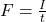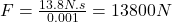## A baseball bat changes the momentum of a ball with an impulse of 13.8 Nᐧs. What is the average force that the bat exerts on the ball if it i

Question

A baseball bat changes the momentum of a ball with an impulse of 13.8 Nᐧs. What is the average force that the bat exerts on the ball if it is in contact with the ball for 0.001 secs.

in progress 0
3 months 2021-08-03T17:11:34+00:00 1 Answers 2 views 0

13800 N

Explanation:

Impulse is the product of average force and time expressed as I=Ft where I is the impulse which results into change in momentum, F is the average force and t is the time of impact. Making F the subject of formula thenSubstituting I with 13.8 N.s and time, t witg 0.001 s then the average force is calculated asTherefore, the average force is equivalent to 13800 N